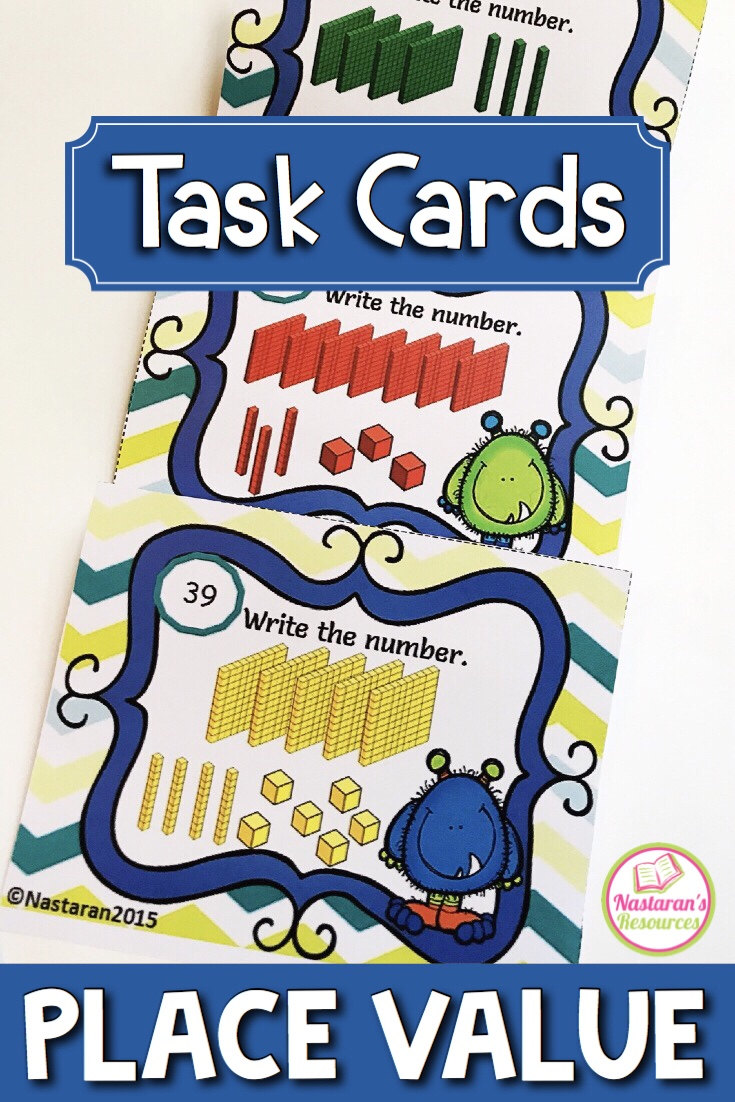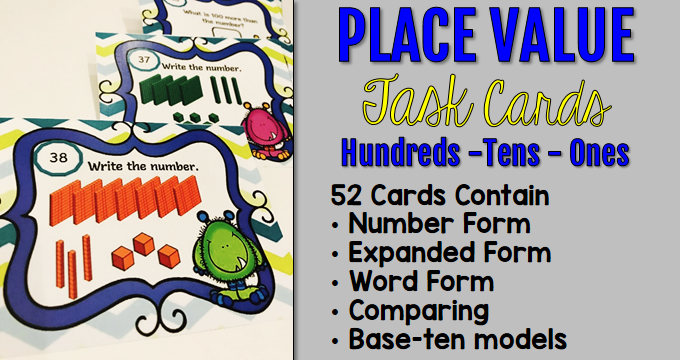You may use these place value task cards to help students to learn or practice their skills on place value in the ones, tens, and hundreds of places. This is perfect for Grade 2 but may also be used as review material for Grade 3 students.

# These Place Value Activities include task cards with this content:

1. Write the number in standard form.
2. What is the value of the underlined digit?
3. Write this number in expanded form.
4. Which digit is in the hundreds place?
5. Which digit is in the tens place?
6. Which digit is in the one’s place?
7. Write this number in word form?
8. Write the digit that is in the place value written.
9. Write the larger number in expanded form.
10. Write the number. (Base-ten models)
11. Draw a model of the number.
12. What number is 100 more than the number?
13. What number is 100 less than the number?

If you like this resource, you may find it HERE or the picture below.

Pin for later :